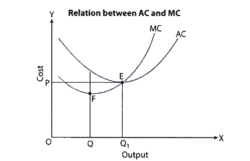# Important Questions Class 11 Economics Part B Unit 3- Producer Behaviour and Supply

Important Questions with Answers for CBSE Class 11 Economics Part B Unit 3 – Producer Behaviour and Supply which is outlined by expert Economic teachers from the latest version of CBSE (NCERT) books

Question 1

Does Total Physical Product increase only when Marginal Physical Product increases?

False, because when Total Physical Product increases Marginal Physical Product decreases but remains positive.

Question 2

What will be the marginal product when the total product is maximum?

Marginal Product will be zero when the total product is maximum.

Question 3

Total Physical Product is derived from Marginal Physical Product by?

(b) Cumulative subtraction

(c) Cumulative product

(d) Cumulative Division

Question 4

What do you mean by production?

Production is the method of producing or developing goods or services in large quantities with the help of various materials.

Question 5

Increase in Total Physical Product indicates that there are increasing returns to a factor. Comment.

No, the total physical product also rises when the returns to a factor decrease.

Question 6

When the returns to a factor decline the marginal and the total product also decline?

False, when returns to a factor decline only Marginal Physical Product declines.

Question 7

Evaluate the marginal product for the following.

 Variable Factor Unit 0 1 2 3 4 5 6 Total Unit 0 5 13 23 28 28 24

 Marginal Product 0 5 8 10 5 0 -4

Question 8

Why does the Average Fixed Cost curve never touch the “x” axis though it lies very close to the x-axis?

The Average Fixed Cost Curve (AFC) never touches the “x” axis though it lies very close to the x-axis because Total Fixed Cost can never be zero.

Question 9

Production function shows a technical relationship between physical input and output of a commodity.

(a) A technological relationship between inputs and cost

(b) The economic relationship between inputs and cost

(c) A technological relationship between inputs and output

(d) A technological relationship between inputs and price

(c) A technological relationship between inputs and output

Question 10

The shape of the Total Physical Product short run is

(a) Inverse U-Shaped

(b) U-Shaped

(c) Hyperbola

(d) V-Shaped

(a) Inverse U-Shaped

Question 11

In the short run Total Product Price changes with the change in which of the following factors.

(a) Economic Cost

(b) Fixed Cost

(c) All the factors

(d) Variable Cost

(d) Variable Cost

Question 12

When TVC is zero at zero levels of output, what happens to TFC or why TFC is not zero at zero level of output?

When TVC is zero at zero levels of output, what happens to TFC or why TFC is not zero at zero levels of output because the fixed cost is to be acquired even at zero levels of output.

Question 13

What is a change in quantity demanded?

It is a change along a demand curve. The change is due to a change in price and quantity of a commodity. The two types of change in quantity demand are Extension in demand and Contraction in demand.

Question 14

Define cost concept. What are the different types of cost?

The spending experienced on different inputs is known as the cost.

The different types of cost are as follows:

Money Cost- Total money spent by a company for manufacturing goods.

Explicit Cost & Implicit Cost- Payment made to an outsider are explicit and cost of self-supplied inputs are implicit cost.

Real Cost- All hard work, discomforts, sacrifices involved in manufacturing a product is called real cost.

Opportunity Cost- This the cost for the next best alternative foregone.

Short Run Cost- Fixed cost- Fixed factors cost

Variable Cost– Variable factor cost

Question 15

Explain the relation between Average Cost and Marginal Cost.